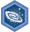## FANDOM

1,895 Pages

6th grade (U.S.): Negative numbersDescription
Achieve mastery in all skills in 6th grade (U.S.): Negative numbers
Details
TypeEnergy point(s) awarded 0
Status Available
Disclosed?
Repeating?

This page contains exercises which pertain to the subcategory 6th grade (U.S.): Negative numbers. Mastering them awards user with the Challenge Patch 6th grade (U.S.): Negative numbers as well.

All items (19)

C
•Comparing absolute values
•Comparing absolute values 2
•Comparing positive and negative numbers on the number line
•Coordinate plane problems in all four quadrants
D
•Distance between points on the coordinate plane
F
•Finding absolute values
G
•Graphing points and naming quadrants
I
•Interpreting absolute value
•Interpreting negative numbers
N
•Negative numbers on the number line
•Negative numbers on the number line without reference to zero
•Number opposites
•Number opposites 2
O
•Ordering rational numbers
•Ordering small negative numbers
P
•Points on the coordinate plane
R
•Rational numbers on the number line
•Reflecting points on the coordinate plane
W
•Writing numerical inequalities